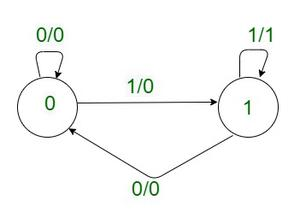Skip to content
Related Articles
GATE | GATE CS 2021 | Set 2 | Question 38
• Last Updated : 23 May, 2021

Suppose we want to design a synchronous circuit that processes a string of 0’s and 1’s. Given a string, it produces another string by replacing the first 1 in any subsequence of consecutive 1’s by a 0. Consider the following example.

```Input sequence : 00100011000011100
Output sequence : 00000001000001100 ```

A Mealy Machine is a state machine where both the next state and the output are functions of the present state and the current input.

The above mentioned circuit can be designed as a two-state Mealy machine. The states in the Mealy machine can be represented using Boolean values 0 and 1. We denote the current state, the next state, the next incoming bit, and the output bit of the Mealy machine by the variables s,t,b and y respectively.

Assume the initial state of the Mealy machine is 0.

What are the Boolean expressions corresponding to t and y in terms of s and b?
(A) t = s+b
y = sb
(B) t = b
y = sb
(C) t = b
y = sb’
(D) t = s+b
y = sb’

Answer: (B)

Explanation:From the above table, we can conclude that : t=b, y=sb.

Hence, option (B) is correct.

Attention reader! Don’t stop learning now. Learn all GATE CS concepts with Free Live Classes on our youtube channel.

My Personal Notes arrow_drop_up